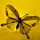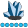## Identify constant columns in a dataset

Hi,

I have a dataset in which there are numeric columns along with character. I wanted to check if any of the columns are having same values thought the table.

Name  num1  num2  num3 num4

a             1         2          3          4

b             1         2         3          4

c             1         3        4          2

If you see the above table num1 is having 1 throughout the table. I have to identify such case in a table

5 REPLIES 5

## Re: Identify constant columns in a dataset

You can use the NLEVELS option of PROC FREQ.

Example:

``````ods select none;
ods output nlevels=nlev;
proc freq data=have nlevels;
run;
ods select all;

data want;
set nlev;
where nlevels=1;
run;``````

The ODS output dataset NLEV also contains counts of missing and non-missing levels, which might be of interest. By using the TABLES statement in the PROC FREQ step you can restrict the set of analysis variables, e.g., to numeric variables:

`tables _numeric_;`

## Re: Identify constant columns in a dataset

Hello,

Do you mean "constant" with missing values not included?
If there are missings and otherwise the column (variable) has a constant value of 1, is that still constant for you ... or not?

For categorical (character) variables I normally use the nlevels option in PROC FREQ.
For numeric variables I check the standard deviation (zero when constant).

A nice CAS-enabled VIYA procedure for this is : PROC CARDINALITY.

Good luck,

Koenwhymath
Lapis Lazuli | Level 10

## Re: Identify constant columns in a dataset

What a beautiful way!whymath
Lapis Lazuli | Level 10

## Re: Identify constant columns in a dataset

You can transform you question to a query:

``````data have;
input name\$ num1 num2 num3 num4;
cards;
a 1 2 3 4
b 1 2 3 4
c 1 3 4 2
;
run;

proc sql noprint;
create table want as
select
count(distinct name)=1 as name,
count(distinct num1)=1 as num1,
count(distinct num2)=1 as num2,
count(distinct num3)=1 as num3,
count(distinct num4)=1 as num4
from have;
quit;``````

The count(distinct var)  can handel both numeric and character variables. Run the program, the result will be one row:

 name num1 num2 num3 num4 0 1 0 0 0

To avoid writing the query statement manual, make it be data-driven:

``````proc contents data=have out=_attr_ noprint;
run;

data _null_;
set _attr_ end=eof;
if _n_=1 then call execute('proc sql noprint; create table want as select');
call execute(ifc(_n_>1,',','')||'count(distinct '||trim(name)||')=1 as '||name);
if eof then call execute(' from have; quit;');
run;``````

By default, the count() function doesn't count missing values, if you want to take missing values into the game, you can convert the variables' value type:

``````proc sql noprint;
create table want as
select
count(distinct cats(name))=1 as name,
count(distinct cats(num1))=1 as num1,
count(distinct cats(num2))=1 as num2,
count(distinct cats(num3))=1 as num3,
count(distinct cats(num4))=1 as num4
from have;
quit;``````

## Re: Identify constant columns in a dataset

Here is a datastep solution:

``````data want;
set have end=done;
array vars(*) _numeric_;
array changes(100) 8 _temporary_;
do _N_= 1 to dim(vars);
changes(_N_)+vars(_N_) ne lag(vars(_N_));
end;
if done;
do _N_= 1 to dim(vars);
if changes(_N_)=1 then do;
vname=vname(vars(_N_));
output;
end;
end;
keep vname;
run;``````
Discussion stats
• 5 replies
• 329 views
• 6 likes
• 5 in conversation